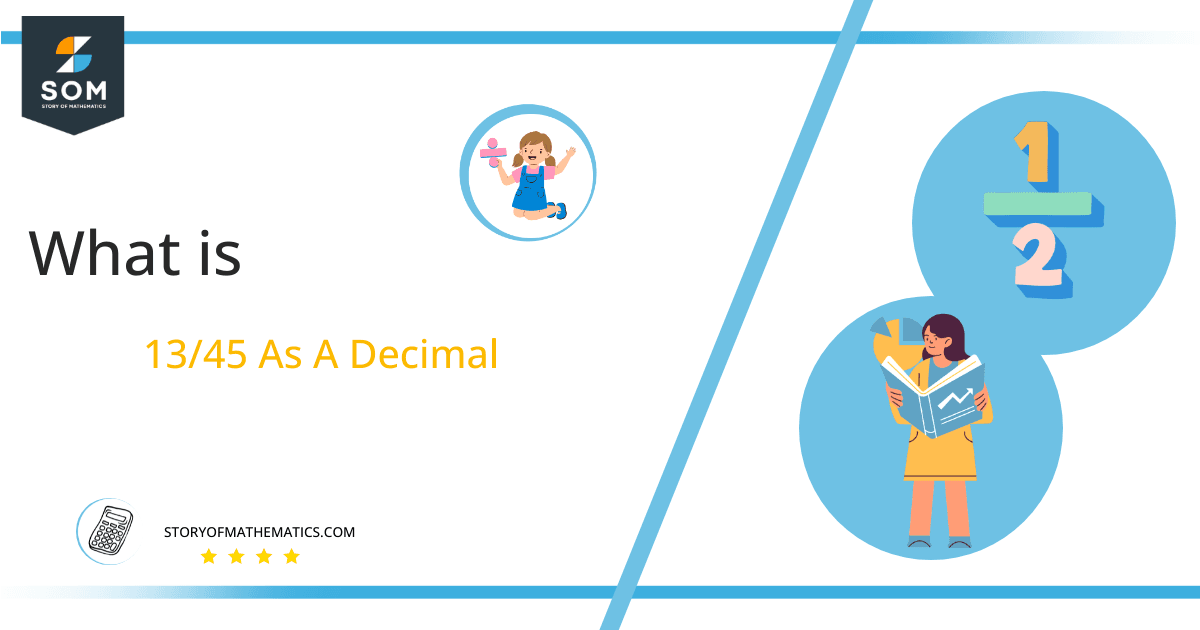# What Is 13/45 as a Decimal + Solution With Free Steps

The fraction 13/45 as a decimal is equal to 0.28888888.

An alternative way to represent a Fraction, which is easier to understand than the fraction is a Decimal and is obtained by dividing components of the fraction.

Here, we are more interested in the division types that result in a Decimal value, as this can be expressed as a Fraction. We see fractions as a way of showing two numbers having the operation of Division between them that result in a value that lies between two Integers.Now, we introduce the method used to solve said fraction to decimal conversion, called Long Division, which we will discuss in detail moving forward. So, let’s go through the Solution of fraction 13/45.

## Solution

First, we convert the fraction components, i.e., the numerator and the denominator, and transform them into the division constituents, i.e., the Dividend and the Divisor, respectively.

This can be done as follows:

Dividend = 13

Divisor = 45

Now, we introduce the most important quantity in our division process: the Quotient. The value represents the Solution to our division and can be expressed as having the following relationship with the Division constituents:

Quotient = Dividend $\div$ Divisor = 13 $\div$ 45

This is when we go through the Long Division solution to our problem.Figure 1

## 13/45 Long Division Method

We start solving a problem using the Long Division Method by first taking apart the division’s components and comparing them. As we have 13 and 45, we can see how 13 is Smaller than 45, and to solve this division, we require that 13 be Bigger than 45.

This is done by multiplying the dividend by 10 and checking whether it is bigger than the divisor or not. If so, we calculate the Multiple of the divisor closest to the dividend and subtract it from the Dividend. This produces the Remainder, which we then use as the dividend later.

Now, we begin solving for our dividend 13, which after getting multiplied by 10 becomes 130.

We take this 130 and divide it by 45; this can be done as follows:

130 $\div$ 45 $\approx$ 2

Where:

45 x  2 = 90

This will lead to the generation of a Remainder equal to 130 – 90 = 40. Now this means we have to repeat the process by Converting the 40 into 400 and solving for that:

400 $\div$ 45 $\approx$ 8

Where:

45 x 8 = 360

So, we have a Quotient generated after combining the two pieces of it as 0.28=z, with a Remainder equal to 40.Images/mathematical drawings are created with GeoGebra.